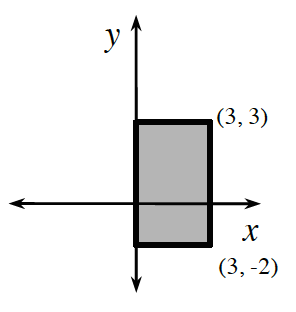### Home > CCA2 > Chapter Ch2 > Lesson 2.1.4 > Problem2-58

2-58.Examine the diagram at right. Imagine spinning the rectangle around the y-axis. Think of a rectangular flap attached to the y-axis so that the rectangle will revolve around the y-axis.  2-58 HW Tool (Desmos). Homework Help ✎.

1. Draw the resulting shape.

After the rotation, the resulting shape is a cylinder.

2. Find the volume of this shape.

$\text{Use the formula for volume of a cylinder, }V=\pi r^{2}h.$

Use the eTool below to help you visualize this shape.
Click the link at right for the full version of the eTool: CCA2 2-58 HW eTool